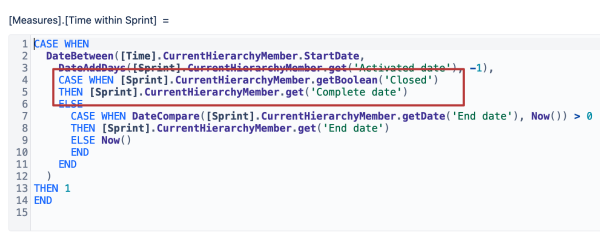# Sprint end day date (time within sprint dosen't work)

i would like to know a way to only show until the sprint end date, had putted time within sprint filter but it doesn’t work it is still showing two day more than the end of sprint i would like to only show util the sprint end date as the image.
i would like to know a way to only show until the sprint end date, had putted time within sprint filter but it doesn’t work it is still showing two day more than the end of sprint i would like to only show util the sprint end date as the image.

Horas gastas measure (hours spent)

``````-- annotations.group = Time tracking
CASE WHEN
DateBetween([Time].CurrentHierarchyMember.StartDate,
DateWithoutTime([Sprint].CurrentMember.get('Start date')),
[Sprint].CurrentMember.get('End date')
)
OR
DateInPeriod(
[Sprint].CurrentMember.get('Start date'),
[Time].CurrentHierarchyMember
)
THEN
([Measures].[Transitions to status],
[Time].CurrentHierarchy.DefaultMember) *
(
DateDiffWorkdays(
DateWithoutTime([Sprint].CurrentMember.get('Start date')),
DateWithoutTime([Sprint].CurrentMember.get('End date'))
) -
DateDiffWorkdays(
DateWithoutTime([Sprint].CurrentMember.get('Start date')),
[Time].CurrentHierarchyMember.StartDate
)
) /
DateDiffWorkdays(
DateWithoutTime([Sprint].CurrentMember.get('Start date')),
DateWithoutTime([Sprint].CurrentMember.get('End date'))
)
END
``````

Hi,

The formula of the Time within the sprint filters the days till the Complete date for closed sprints. You can check the formula behind it:If that is not what you wish to have, you can adjust this formula to always work with the End date instead of the Complete date.

Let me know if that explains the behavior of the report or if further guidance is needed.

Kindly,
Janis, eazyBI support